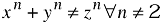# equation

\$Revision: 1.3 \$

\$Date: 2002/06/12 11:18:09 \$

equation — A displayed mathematical equation

## Synopsis

### Content Model

```equation ::=
(blockinfo?,
(title,titleabbrev?)?,
(informalequation|
(alt?,
(graphic+|mediaobject+))))
```

### Attributes

Common attributes

 Name Type Default label CDATA None

## Description

An Equation is a formal mathematical equation (with an optional rather than required title).

If the MathML Module is used, Equation can also contain the mml:math element.

### Processing expectations

Formatted as a displayed block. For an inline equation, use InlineEquation.

Processing systems that number equations or build a table of equations at the beginning of a document may have difficulty correctly formatting documents that contain both Equations with Titles and Equations without Titles. You are advised to use InformalEquation for equations without titles.

### Future Changes

In some future version of DocBook, probably V5.0, even though the change has not yet been announced, the Title on Equation will be required. For equations without titles, use InformalEquation.

### Parents

These elements contain equation: .

### Children

The following elements occur in equation: .

## Attributes

label

Label specifies an identifying string for presentation purposes.

Generally, an explicit Label attribute is used only if the processing system is incapable of generating the label automatically. If present, the Label is normative; it will used even if the processing system is capable of automatic labelling.

## Examples

```<!DOCTYPE equation PUBLIC "-//OASIS//DTD DocBook XML V4.1.2//EN"
"http://www.oasis-open.org/docbook/xml/4.1.2/docbookx.dtd">
<equation><title>Fermat's Last Theorum</title>
<alt>x^n + y^n &ne; z^n &forall; n &ne; 2</alt>
<graphic fileref="figures/fermat.png"/>
</equation>
```

Equation 1. Fermat's Last Theorum